Original Article

Journal of Biosystems Engineering. December 2018. 379-385
https://doi.org/10.5307/JBE.2018.43.4.379

# MAIN

• Introduction

• Materials and Methods

• Results and Discussion

•   Component design

•   Construction and performance test

• Conclusions

• Conflict of Interest

Introduction

Onions for postharvest handling in Korea are mainly treated using 20 kgf net packages, thus resulting in high-handling costs and low-work efficiency. Park et al. (2013; 2015) has identified the problems of 20 kgf net- package-based handling, including the (1) decreased efficiency because more net packaging should be performed before shipment, (2) increased risk of compression damage, and the (3) difficulty to remove the bulbs decayed during storage. Given the reduction of the labor pool and the increasing wages owing to the rapid population aging in the rural Korean society, it is urgently required to develop a more efficient postharvest system that could replace the current 20 kgf net-package-based handling system.

Park et al. (2009) have developed a postharvest bulk handling system which can grade, transport, and store onions in bulk state to replace the 20 kgf-net package- based handling. In the postharvest bulk handling system for onions, onions are safely loaded on the box pallet and various processing steps are carried out in the bulk state. Therefore, unhealthy onions can be easily sorted out during storage. To develop this system, research studies have been conducted on the frictional characteristics and airflow resistance of onions, and on the design of a box pellet (Park et al., 2013; Park et al., 2015).

The purpose of this study is to determine the design parameters and operating conditions of the auto-dump system that constitutes a key component of the postharvest bulk handling machinery system for onions, and to evaluate its performance using a prototype auto-dump system.

Materials and Methods

The schematic diagram of postharvest bulk handling machinery system (Park et al., 2009) is shown in Figure 1. The system includes a box pallet for batch handling, a device (an automatic feed hopper with a feeding rate control device, and a belt conveyor with a chute) for temporary stacking and feeding onions from bulk bags to the system, an auto-dump for lifting and descending the pallet, an automatic empty-pallet feeding device, and an automatic pallet unloading device.

Kinematic analysis and computer simulation techniques were conducted to evaluate the operating parameters and conditions of the auto-dump.

The auto-dump (item (5) in Fig. 1) assisted the lifting of the empty-box pallet (L×W×H=1,300×1,100×1,600 mm, capacity=1 ton) (Park et al., 2015) to a predetermined angle and the lowering of the loaded onion pallet. Additionally, a hydraulic cylinder was used for accurate velocity control and repeatability. It is important to analyze the relationship between the angular velocity of the pallet mounting device and the hydraulic cylinder velocity for the determination of the operating conditions of the auto-dump and control of the bulk handling machinery system.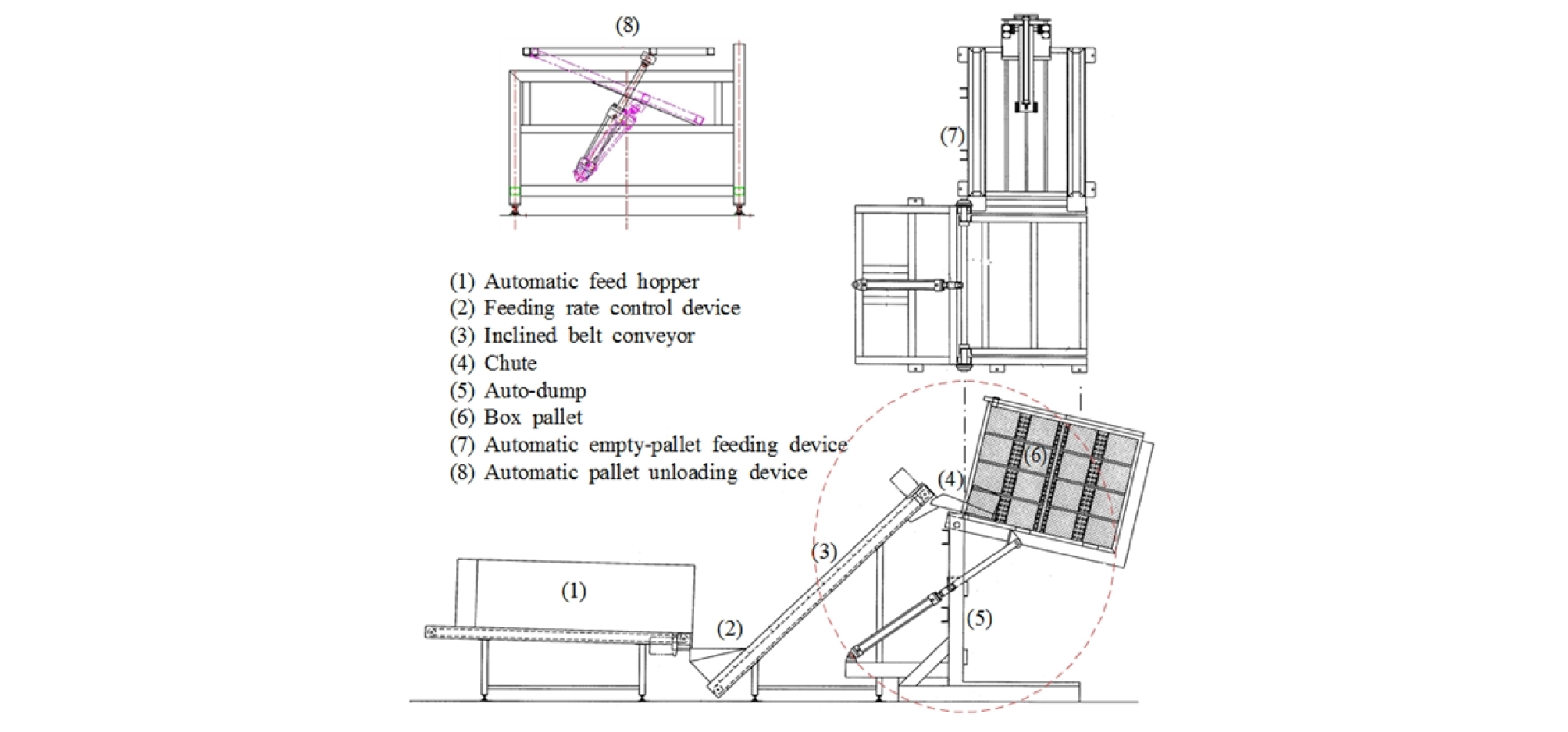##### Figure 1.

Schematic of postharvest bulk handling machinery system.

The velocity at the auto-dump was expressed in the r–θ coordinate system (Fig. 2) using 𝜈r in equation 1 and 𝜈𝛳 in equation 2, where 𝛼=𝛽-𝛳 and $\nu =\overline{)AC}\stackrel{˙}{\beta }$.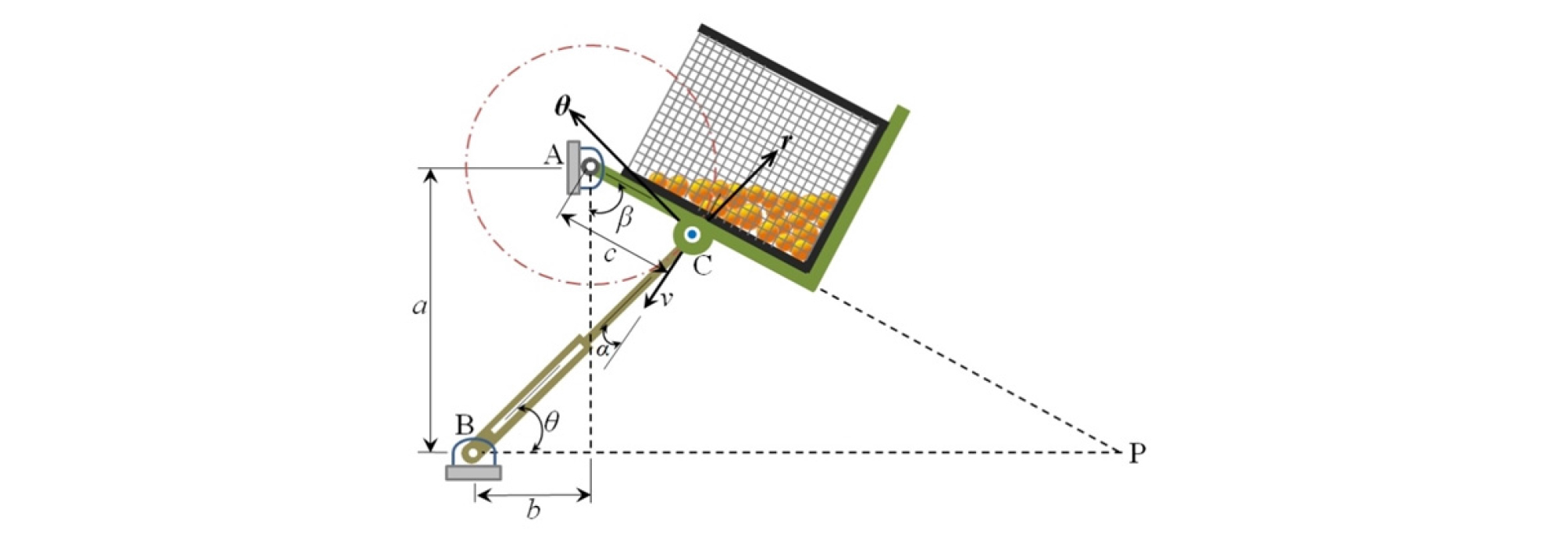##### Figure 2.

Auto-dump mechanism.

 $$\nu_r=\dot r=\nu cos\mathit\;\alpha\mathit=c\overset{\mathit˙}{\mathit\beta}\mathit{cos}\left(\mathit\beta\mathit-\mathit\theta\right)$$ (1)
 $$\nu_\theta=r\dot\theta=\nu sin\mathit\;\alpha\mathit=c\overset{\mathit˙}{\mathit\beta}sin\left(\mathit\beta\mathit-\mathit\theta\right)s$$ (2)

In addition, using trigonometric functions, the tangent of angle θ can be expressed according to equation 3.

 $$\tan\theta=\frac{a-c\cos\beta}{b+c\sin\beta}$$ (3)

After considering the lifting stability (for example, vertical separation distance of the hydraulic cylinder from the ground) and the turning radius of the auto- dump with the box pallet, the vertical distance between both hinges (a in Fig. 2) and the maximum rotating angle (β in Fig. 2) of the auto-dump were set to 1,345 mm and 77°, respectively. Both ends of the hydraulic cylinder were connected to the hinge by revolute joints. However, if the head end of the hydraulic cylinder is placed on point B to minimize the changes of angle θ formed by a horizontal line, the piston rod of the hydraulic cylinder is capable of a linear translation with a minimal rotational movement. As a result, the piston rod can only be subjected to the axial load so that a more stable operation can be secured.

Using equation 3, a three-dimensional response surface analysis was performed with the setting angle of the cylinder (θ) as a dependent variable, and the installation distance (b) of the hydraulic cylinder and the rotating angle of the auto-dump (β) as the independent variables. Herein, position C on the auto-dump was predetermined to be 642 mm from the upper hinge (A) in consideration of the center of gravity of the auto-dump with the box pallet. Additionally, the installation distance (b) of the hydraulic cylinder was directly related to the stroke of the hydraulic cylinder. That is, as the installation distance (b) increases, the stroke of the hydraulic cylinder must be large. As shown in Figure 3, when the installation distance (b) was 1,008 mm, the angular deviation of the setting angle (θ) on the rotation of the auto-dump was relatively small and its mean value was 32.6° (standard deviation =±1.9°).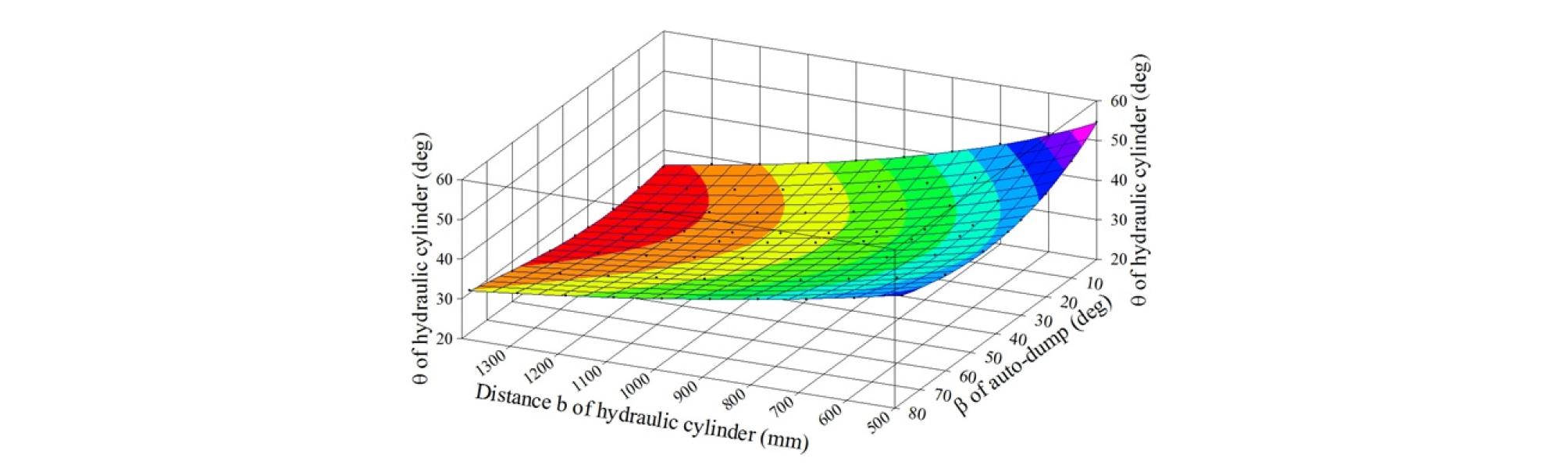##### Figure 3.

Response surface for the setting angle (θ) of the hydraulic cylinder as a function of installation distance (b) of the hydraulic cylinder and the rotating angle (β) of the auto-dump.

When the results listed above are used, the stroke control range of the hydraulic cylinder is ∆l=743 mm when the rotating angles of the auto-dump (β) are 77° and 0° (equation 4).

 $$I=\frac{b+c\sin\beta}{\cos\theta}$$ (4)

where b=1,008 mm, c=642 mm, θ=32.6°, and 77°≥β≥0°.

When the rotating angle of the auto-dump (β) was changed from 77° to 0°, the changes of the angular velocity at various velocities of the hydraulic cylinder were analyzed with equation 1 and are illustrated in Figure 4. The changes of the angular velocity of the auto-dump decreased when the velocity of the hydraulic cylinder was reduced, and the minimum was observed when the rotating angle of the auto-dump was approximately 33°.##### Figure 4.

Response surface for the angular velocity (dβ/dt) of the auto-dump as a function of velocity (v) of the hydraulic cylinder and the rotating angle (β) of the auto-dump.

Figure 5 shows the rotational time of the auto-dump at a rotating angle of 77° as a function of the velocity of the hydraulic cylinder. If 90% of the onions could be filled in box pallets during the rotation of the auto-dump and in a total time of less than 4 min (240 s), the rotational time (working time) becomes equal to 216 s (= 240 s × 0.9). Correspondingly, the velocity of the hydraulic cylinder and the mean angular velocity are 3.59 mm/s and 0.36°/s, respectively (Fig. 5). We assumed that it took 4 min for one worker to fill the box pallet with one ton of onions.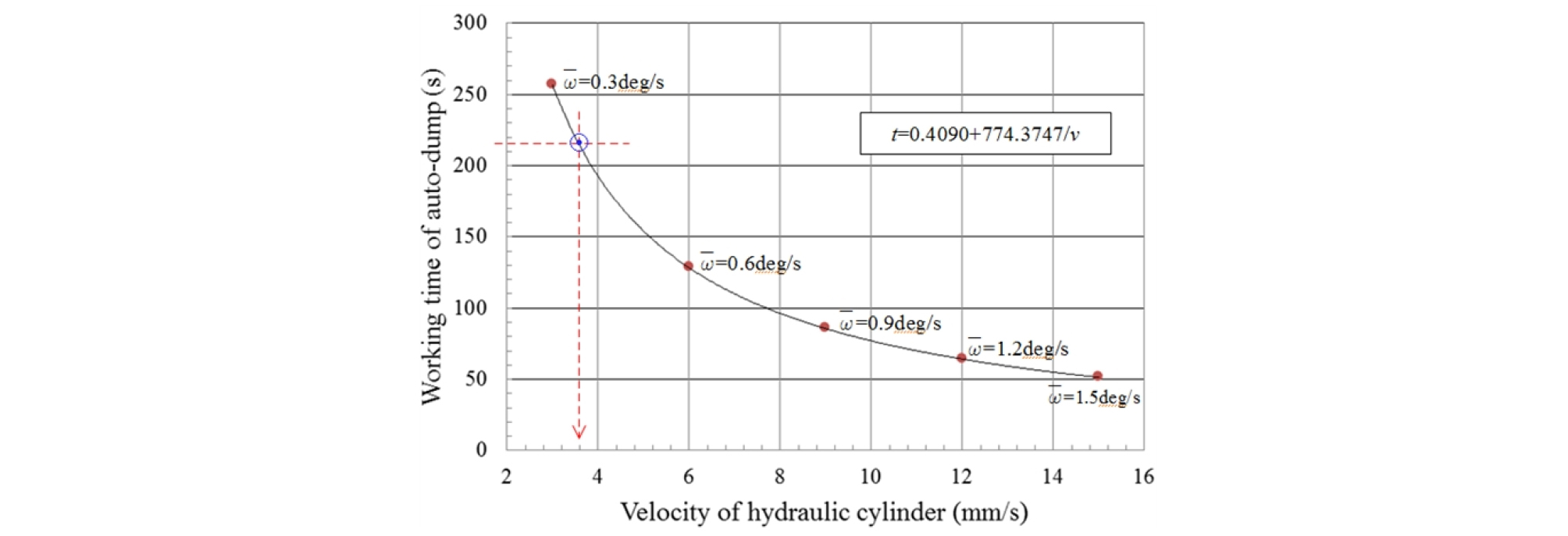##### Figure 5.

Working time of the auto-dump versus velocity of the hydraulic cylinder.

A computer simulation for the auto-dump was conducted with the analytical analysis. Each component was briefly modeled with a commercial 3D design tool Each component was briefly modeled with a commercial 3D design tool, CATIA (ver. 5 R18, Dassault Systems, Waltham, MA, USA), and was then assembled using Adams (ver. R2, MSC Software, Newport Beach, CA, USA) with the boundary conditions. with the set boundary conditions.

A preliminary model of the auto-dump is illustrated in Figure 6. The simulation model was constructed with three revolute joints (#1, #2, and #3), which enabled rotational motion in one direction (z–axis), and the use of a translational joint, which allowed rectilinear motion in the axial direction of the cylinder (Fig. 7). When the hydraulic cylinder moved backward at a velocity of 3.59 mm/s slanting with the horizontal line at the revolute joint #3, the pivoting frame of the auto-dump yielded a rotational motion in the clockwise direction from 77° to 0° at the revolute joint #1.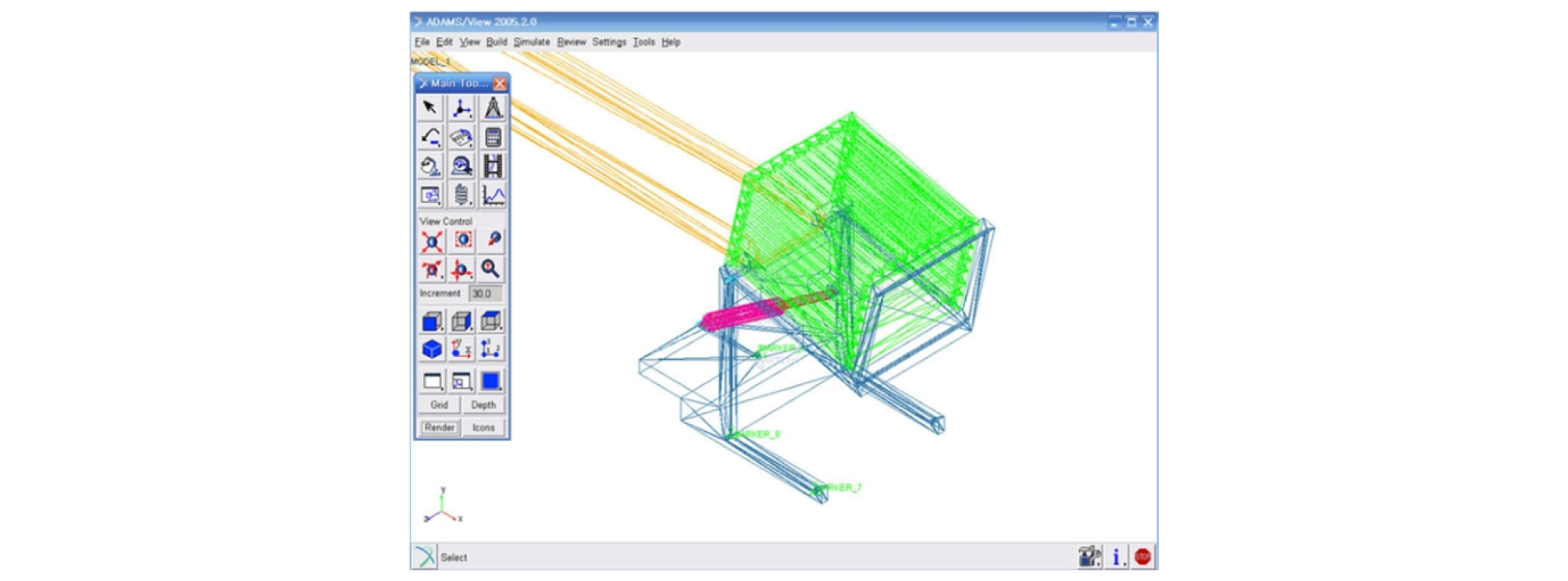##### Figure 6.

Modeling of the auto-dump system.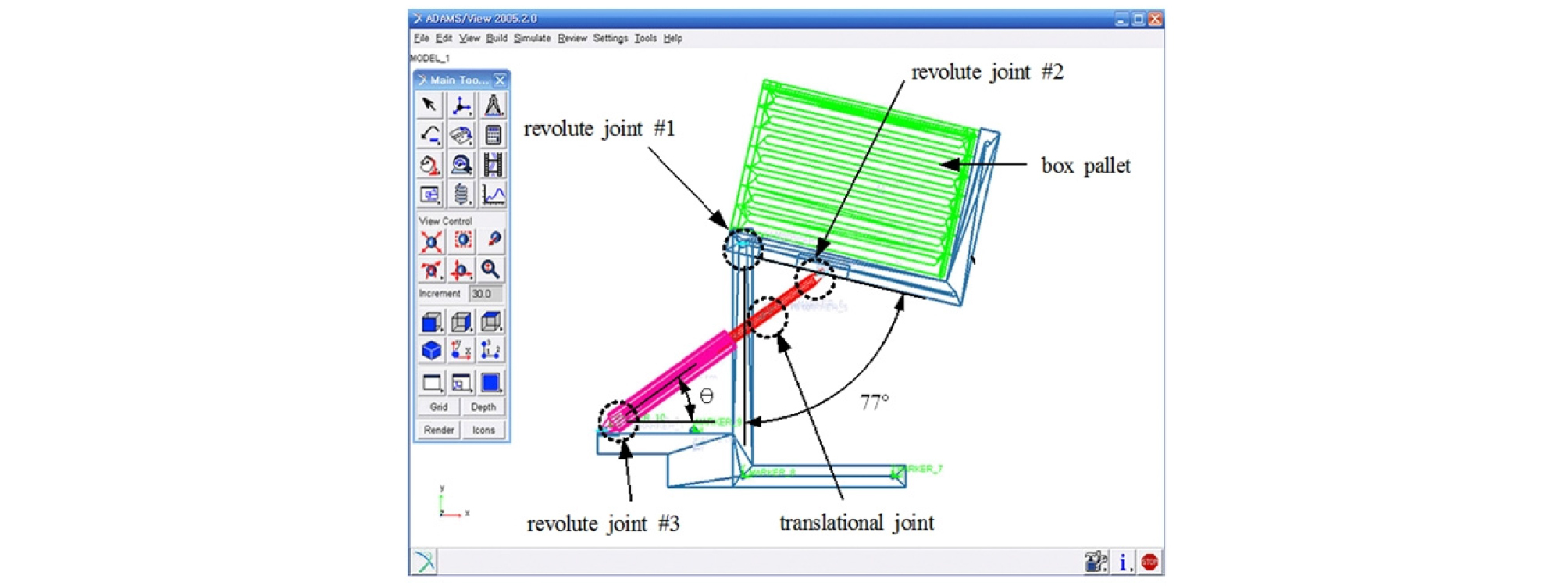##### Figure 7.

Simulation model based on the application of the boundary conditions.

The pivotal frame yielded a constant angular movement at 77° at the revolute joint #1, and the hydraulic cylinder elicited a minimum angle of 31° at 115 s, and a maximum angle of 37° at 216 s at the revolute joint #3 (refer to the line of “θ of the hydraulic cylinder” in Fig. 8). The mean value was 32.5° (standard deviation=±1.5°) and the displacement caused by wobbling was insignificant.

In addition, when the initial angular velocity was 0.39°/s, its minimum and maximum velocities were 0.32°/s at 115 s and 0.44°/s at 216 s, while the auto- dump was rotating (refer to the red line in Fig. 8). These values were consistent with the results of the three- dimensional surface response analysis.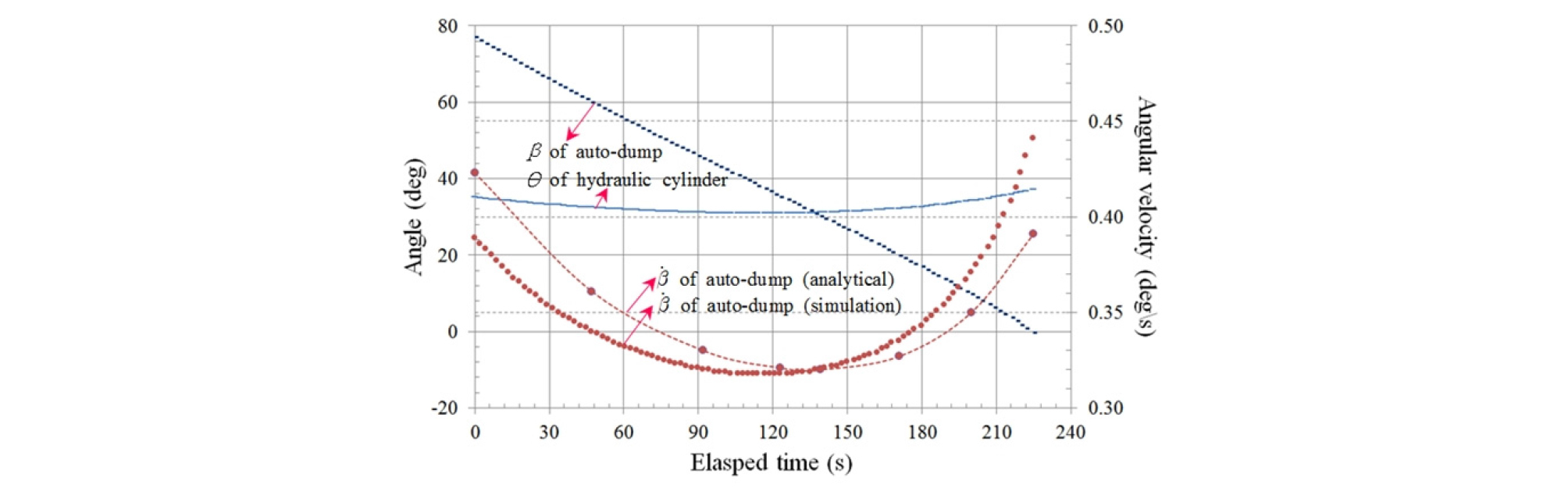##### Figure 8.

Rotating angle and angular velocity as a function of elapsed time according to the simulation results.

Results and Discussion

Component design

The capacity of the hydraulic cylinder is one of the most important factors in designing an auto-dump. The moving part of auto-dump was regarded as an assembly and consisted of a box pallet, a pallet mounting device (a pivoting frame), and the stacked onions. Its motion was considered to be planar and was limited to the two- dimensional plane. The center of gravity at the auto- dump’s rotational angles and the force which acted on the hydraulic cylinder were analyzed according to this mechanism.

The weight of an empty pallet was 1,177 N (Park et al., 2015), and the total weight of the auto-dump was 2,450 N when the weight per unit length of the pivoting frame (pallet mounting device) (L-shape, 1,750×1,312 mm) was 800 N/m.

Owing to the irregular shape and internal friction of the onions, the onions stacked on the box pallet are oriented at an angle with respect to the horizontal plane, as shown in Fig. 9(a). Assuming that the inclination angle is 20°, which is the angle of repose of the onion (Park et al., 2013; Sitkei, 1986), the onions will cover the entire bottom surface of the box pallet when the rotating angle of the auto dump reaches 30° (Fig. 9). Accordingly, the stacking patterns of onions were different at different rotational angles because one ton of onions was stacked when the auto-dump was rotating along a triangular path when 77°>β≥35°, a trapezoidal path when 35°>β>0°, and along a rectangular path when β=0°.##### Figure 9.

Stacking patterns and center of gravity of the assembly at various rotating angles of the auto-dump.

Figure 10 shows the center of gravity of the assembly at various rotating angles as a function of the weight of the onions that are being stacked. The center of gravity of the assembly was calculated by applying the principle of moments to the each finite element of the assembly. The bulk density of onions applied in the calculation was 541.80 kg/m3 (Park et al., 2013).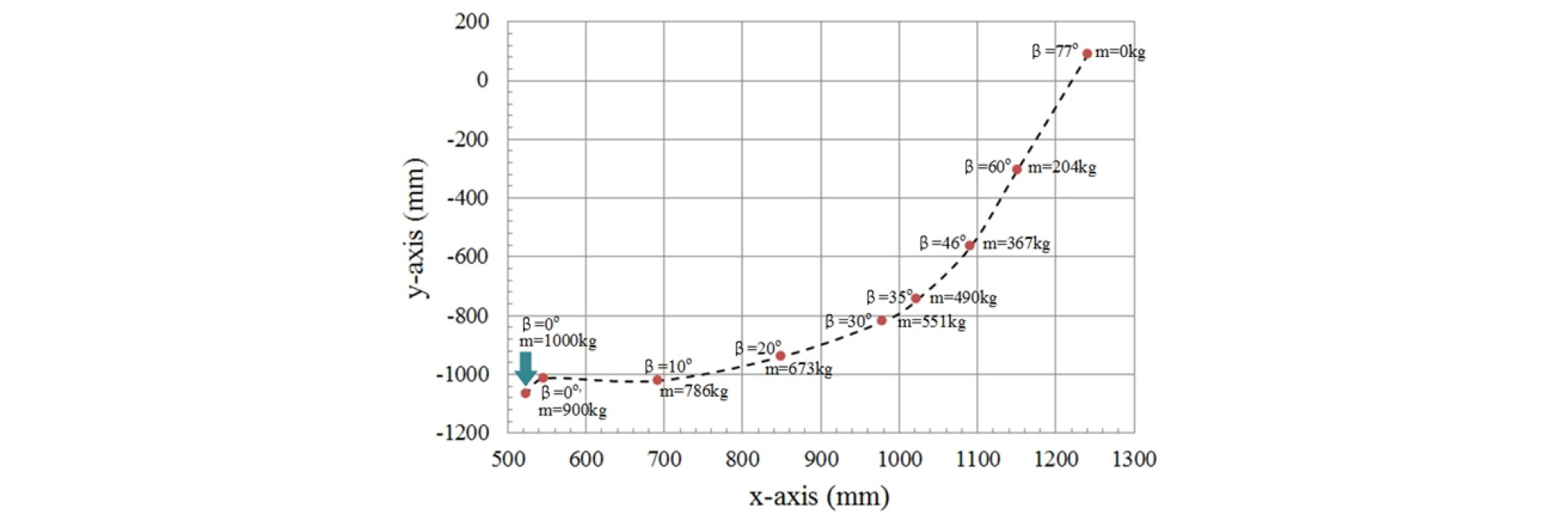##### Figure 10.

Center of gravity of the assembly as a function of the rotating angle of the auto-dump for different weights of stacked onions.

The position of the center of gravity in the assembly was changed rapidly during the rotation and slowed down at the bottom left. Almost no movement was observed in the vertical (y–axis) direction, and the rotating angles were less than approximately 17°. In addition, the last 10% of onions (from 900 kg to 1,000 kg) were stacked when the rotation was completed (see the red arrow in Fig. 10).

The force acting on the hydraulic cylinder was determined by considering the position of the center of gravity and the static equilibrium condition, as shown in Figure 11. When the auto-dump operates, onions are stacked at a feeding rate of $\stackrel{˙}{m}=250\mathrm{kg}/\mathrm{min}$ from an angle of 77°. The force acting on the hydraulic cylinder increased as the rotating angle decreased to 23°, whereby the maximum force observed was 14 kN. The point indicated by the arrow in Figure 11 illustrates the force exerted during the filling (of the remaining 10%) of the onions in the vertical state.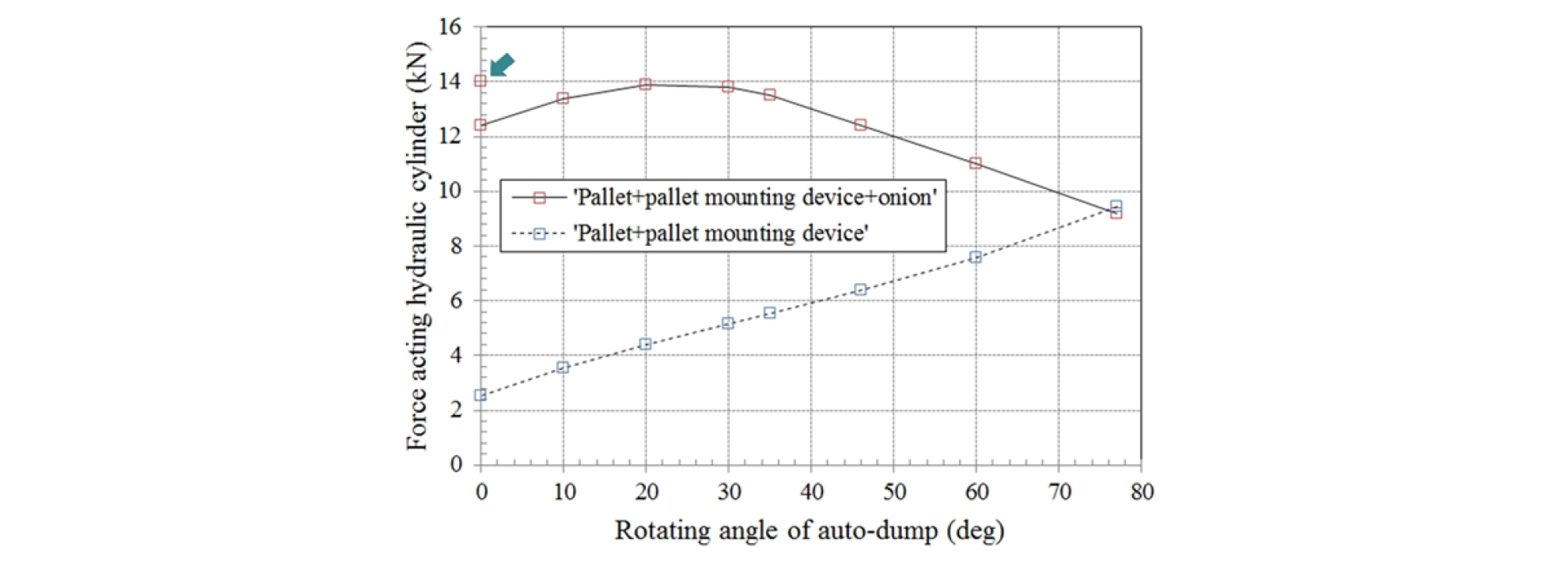##### Figure 11.

Force acting on the hydraulic cylinder at various rotating angles of the auto-dump.

It is essential to determine the optimum diameter and stroke of the cylinder for proper loading in the design process of the hydraulic cylinder. The maximum force acting on the front end of the piston rod of the hydraulic cylinder was 14 kN, and the normal working pressure of the hydraulic cylinder was 9.81 MPa. Because the load was close to the static load, the inner diameter (69 mm) was calculated using Equation 5 by considering a factor of safety of two. The diameter of the cylinder, however, was determined to be 80 mm based on the Standards of Private Sectors (KTC, 2015), and the diameter of the piston rod was determined to be 45 mm because type B was selected owing to the large piston stroke of the actuating mechanism.

 $$F=A\times P\times\beta$$ (5)

where A=area of piston (m2), P=working pressure (Pa), and β=effective efficiency (80%).

In addition, the buckling strength of the piston rod should be considered. The critical load (Pcr) was calculated using Euler’s equation (equation 6) because the slenderness ratio was more than 90 (Lee, 2007).

 $$\begin{array}{l}k=\sqrt{\frac IA}=\sqrt{\frac{\pi d^4}{64}\times\frac4{\pi d^2}}=\frac d4=0.0113m,\\\lambda=\frac Lk=\frac2{0.0113}=117>90\;P_{cr}=\frac{n\pi^2EI}{L^2}\times\frac1S\end{array}$$ (6)

where k=radius of gyration of area (m), I=moment of inertia (m4), λ=slenderness ratio, L=distance between both ends of the cylinder (1.94 m based on equation 4), n=modulus of end (1 in the case of the hinged end (by pin) at both ends), E=Young’s modulus (205 GPa on S45C) (Soyull and Soyull, 2006), and S=safety factor (higher than four).

The moment of inertia of the tube of the hydraulic cylinder was larger than that of the piston rod. However, in general, the moment of inertia of the piston rod was used to simplify the calculation and consider the safety of the design. Therefore, the critical load (Pcr) was calculated using equation 5 as follows,

 $$I=\frac{\mathrm\pi d^4}{64}=\frac{\pi\times0.045^4}{64}=2.0119\times10^{-7}\mathrm m^4$$ (7)
 $$\begin{array}{l}P_{cr}=\frac{n\pi^2EI}{L^2}\times\frac1S\\=\frac{1\times\mathrm\pi^2\times\left(2.05\times10^{11}\right)\times\left(2.0119\times10^{-7}\right)}{1.94^2}\times\frac14\\=27,012\mathrm N\end{array}$$ (8)

The maximum force acting on the hydraulic cylinder ranged between 14 kN and 27.0 kN. This was less than the critical load and ensured the safety of this design. In addition, 80B–800 (working pressure 70–140 MPa) was determined by the Standards of Private Sectors (KTC, 2015). Attaining uniform motion from 77° to 0° and a precise mounting of the empty-pallet were important in designing the auto-dump. Therefore, a double-acting cylinder with unit counter balance was selected for an easier control of velocity and corrected motion.

Construction and performance test

Figure 12 shows the detailed design of the auto-dump, and its 3D model and performance test are shown in Figures 13 and 14, respectively. The performance test for the prototype of the auto-dump was conducted to evaluate the stacking pattern of the onion and the stability of the system when the box pallet rotated from 77° to 0° while maintaining the feeding rate of the onion and the velocity of the hydraulic cylinder at $\stackrel{˙}{m}=250\mathrm{kg}/\mathrm{min}$ and 3.59 mm/s, respectively.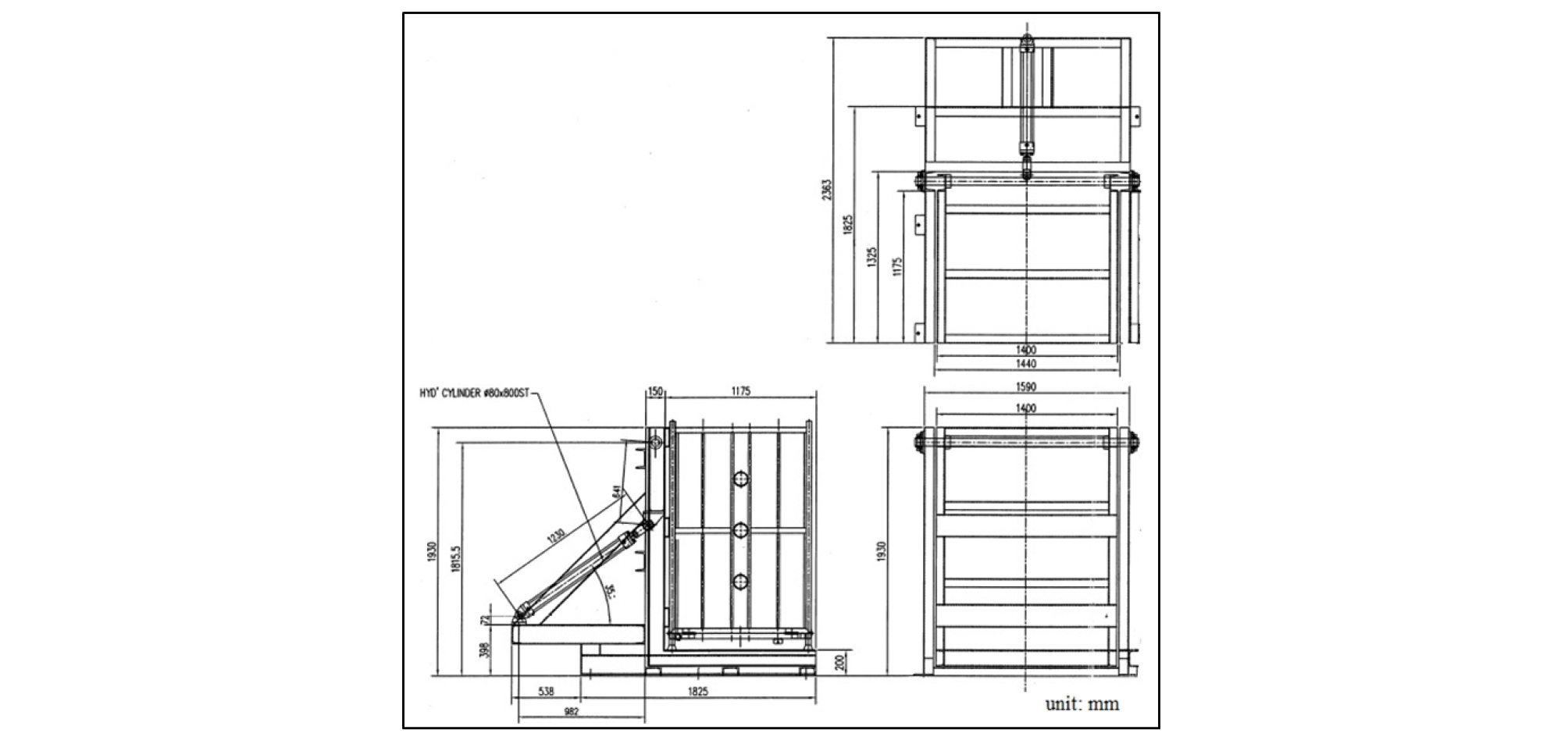##### Figure 12.

Drawings of the auto-dump.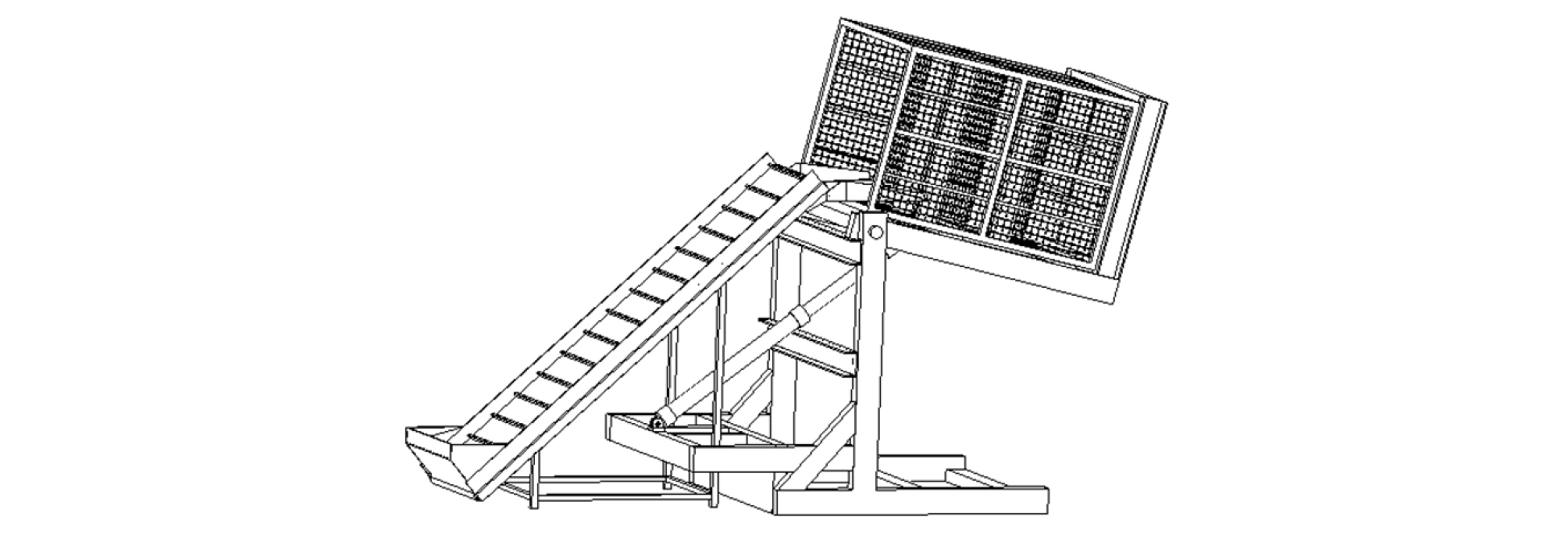##### Figure 13.

3D modeling based on the detailed design drawings for the auto-dump.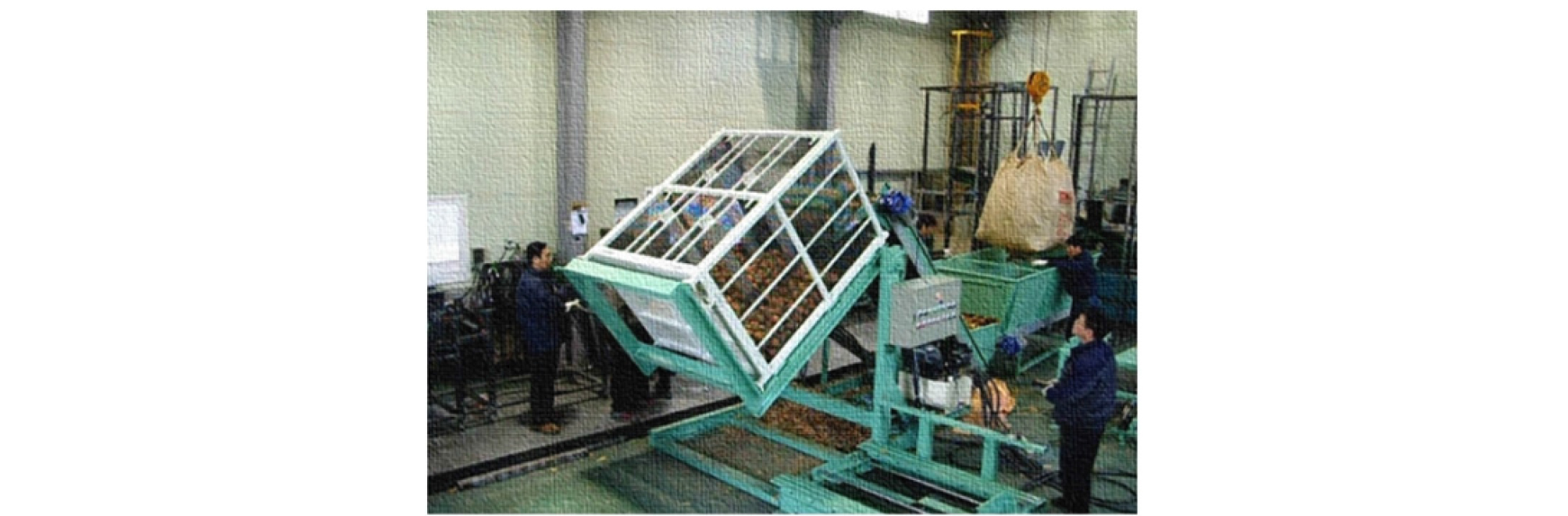##### Figure 14.

Performance test with the auto-dump prototype.

During the operation of the auto-dump, the stacking pattern of the onion was well formed at 20° near the angle of repose at the beginning of the rotation, but the stacking pattern was somewhat nonuniform owing to the vent pipe installed in the box pallet. However, the operating status of the auto-dump during operation was evaluated to be very stable in terms of its vibration and stiffness responses.

To improve the stacking pattern of onions, two control methods were evaluated: one was the control of the rotational speed of the auto-dump, and the other was the control of the feeding rate of the onion. Experimental analyses of both methods showed that the two-stage method used to control the feeding rate of the onion was improved compared to the two-stage control method of the rotational speed of the auto-dump. Additionally, it is shown that the method used to control the feeding rate of onions is more advantageous compared to the method of controlling the rotational speed of the auto-dump in terms of the control of the overall system (postharvest bulk handling machinery system).

Conclusions

In this study, the kinematic analyses and simulations of the auto-dump—the key component of the postharvest bulk handling machinery system for onions—were conducted to fill one ton of onions with the use of a box pallet. The capacity, installation angle, and position of the hydraulic cylinder were analyzed to achieve linear translation with minimal rotational movement during the rotation of the auto-dump. The proper installation angle and velocity of the hydraulic cylinders were 32.6° and 3.59 mm/s, respectively. In addition, the average angular velocity of the auto-dump was 0.36°/s. When one ton of onions was set to be filled into the box pallet in 4 min, it was considered appropriate to fill 90% of the pallet while the auto-dump was rotating, and 10% after the rotation stopped. Additionally, for the uniformity of the stacking pattern, a two-stage control method for the feeding rate of onions was preferred. As a result of the performance test with the auto-dump prototype, the control of the feeding rate of onions improved in terms of the uniformity of the stacking pattern and the control of the entire system compared to the control of the rotational speed of the auto-dump.

Conflict of Interest

The authors have no conflicting or other interests.

## Acknowledgements

This work was supported by a two-year Pusan National University research grant.

## References

1
KTC. 2015. SPS-KTC-B6370-0002: Hydraulic cylinders. Gunpo, Republic of Korea: Korean Testing Certificate.
2
Lee, J.G. 2007. Hydraulic Systems. Seoul, Republic of Korea: Kijeon Publishing Company (in Korean).
3
Park, J. M., D. S. Choi, S. H. Kwon, S. W. Chung, S. G. Kwon, W. S. Choi and J. S. Kim. 2015. Development of a box pallet for post-harvest bulk handling system on onions. Agricultural Mechanization in Asia, Africa, and Latin America (AMA) 46(4): 22-27.
4
Park, J. M., G. S. Kim, S. H. Kwon, S. W. Chung, S. G. Kwon, W. S. Choi, J. S. Kim, M. Mitsuoka, E. Inoue and T. Okayasu. 2013. Development of post-harvest bulk handling system of onions (I) - Characteristics of friction and airflow resistance. Journal of the Faculty of Agriculture Kyushu University 58(1): 87-92.
5
Park, J.M. 2009. Development of a post-harvest bulk handling system. Report number 107029-2. Suwon, Republic of Korea: Rural Development Administration (RDA) (in Korean).
6
Sitkei, G., 1986. Mechanics of agricultural materials, 8nd ed. New York, NY, USA: Elservier.
7
Soyull, B. and D. Soyull. 2006. Handbook for Mechanical Design. 5th ed. Seoul, Korea: Daekwang Publishing Company (in Korean).